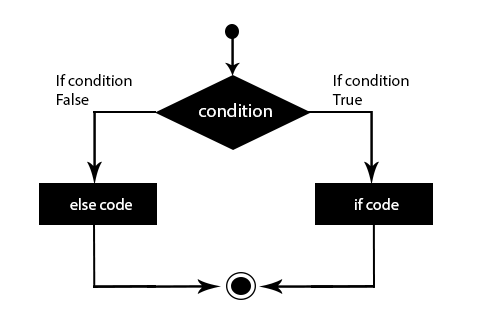## Python Tutorials: Learn Python Statements in Detail

##### In this part of Learning Python we Cover how to use Statements in python
Written by |19-Oct-2020 | 0 Comments | 635 Views

In python, there are two types of statements IF or ELSE. It can be explained by the example, “A father told his son to go to his room and search for his glasses if he finds his glasses than bring those glasses to him; otherwise, go to mom and asked her about the glasses.” The same is the property of if-else in python. The basic syntax of the if-else statement is the following:

Syntax:

 If( condition ):     #statement else:     #statement

The above-defined syntax is compulsory for the if-else statement. There must be 4 tabs indentation after the condition, and there must be a colon after if and else.

Some of the example codes are following, which make it clearer.

#Case1

 string = "Pooh"   if(string=="Pooh"):     print("String Matched") else:     print("String Not Matched")

In the above case, the string is actually equal to pooh, so if the condition will be printed.

#Case2

 string = "Alladin" if(string=="Pooh"):     print("String Matched") else:     print("String Not Matched")

In case 2 string is not matched so else statement will be printed.

Finding a word in the string:

suppose we have a long string, and we want to know that particular word is present in a string or not for that we can use if statements. If that word is present in that string, then it will say I found else not found.

 string = '''Python is one of the growing programming languages according to StackOverflow, especially in the field of data science and machine learning'''   if 'machine learning' in string:     print('Yes it is there') else:     print('not present')

The in keyword search that particular argument in a string or maybe in a list.

We can negate by using not keyword before in keyword as following:

 string = '''Python is one of the growing programming languages according to StackOverflow, especially in the field of data science and machine learning'''   if 'Java' not in string:     print('Not present') else:     print('present')

Statements with bool operators:

We can also verify the conditions by using the bool operator in a condition section. Following is the example which clarifies the concept:

 friend1 = "Robert" friend2 = "Peter"   if((friend1=="Robert")and(friend2=="Peter")):     print("Both friends matched") else:     print("not matched")

Elif Statement:

We can use multiple if statements by using the elif keyword for this:

Following is the example of using the elif keyword:

 friend1 = "Robert" friend2 = "Peter"   if((friend1=="Robert")and(friend2=="Norton")):     print("If statement run")   elif((friend1=="Robert")and(friend2=="Peter")):     print("Elif statement run")   else:     print("else run")

As we see, the condition of the elif statement is true, so the output will be “Elif statement run.”

We can represent the flow diagram of the if-else statement as follows:As we can see, the code starts it enter into the condition where it has to make a decision. If the condition is true, then the “if” block is executed; otherwise, “else” code will be executed.